# 2.1 Defining Functions

You have been composing code that calls Python functions since the beginning of this booksite. In this section, you will learn how to define and call your own functions. Functions support a key concept that will pervade your approach to programming from this point forward: Whenever you can clearly separate tasks within a computation, you should do so.

## Using and Defining Functions

The effect of calling a Python function is easy to understand. For example, when you place `math.sqrt(a-b)` in a program, the effect is as if you had replaced that code with the return value that is produced by Python's `math.sqrt()` function when passed the expression `a-b` as an argument. If you think about what the system has to do to create this effect, you will see that it involves changing a program's control flow.

You can define a function using a `def` statement that specifies the function signature, followed by a sequence of statements that constitute the function. For example, harmonicf.py defines a function named `harmonic()` that takes an argument `n` and computes the `n`th harmonic number (as described in Section 1.3). It also illustrates the typical structure of a Python program, having three components:

• A sequence of `import` statements
• A sequence of function definitions
• Arbitrary global code, or the body of the program

Python executes the global code when we invoke the program by typing `python harmonicf.py` on the command line; that global code calls the `harmonic()` function defined earlier. (For purposes of illustration, we have made the user-interaction part of the program a bit more complicated than in Section 1.3.)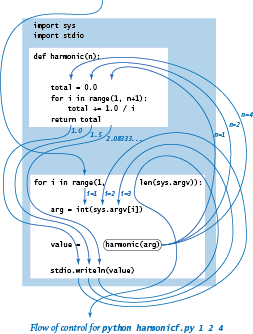### Control flow.

The diagram shown at the right illustrates the flow of control for the command `python harmonicf.py 1 2 3`. First, Python processes the `import` statements, thus making all of the features defined in the `sys` and `stdio` modules available to the program. Next, Python processes the definition of the `harmonic()` function at lines 4 through 8, but does not execute the function — Python executes a function only when it is called. Then, Python executes the first statement in the global code after the function definition, the `for` statement, which proceeds normally until Python begins to execute the statement `value = harmonic(arg)`, starting by evaluating the expression `harmonic(arg)` when `arg` is 1. To do so it transfers control to the `harmonic()` function — the flow of control passes to the code in the function definition. Python initializes the "parameter" variable `n` to 1 and the "local" variable `total` to 0.0 and then executes the `for` loop within `harmonic()`, which terminates after one iteration with total equal to 1.0. Then, Python executes the `return` statement at the end of the definition of `harmonic()`, causing the flow of control to jump back to the calling statement `value = harmonic(arg)`, continuing from where it left off, but now with the expression `harmonic(arg)` replaced by 1.0. Thus, Python assigns 1.0 to `value` and writes it to standard output. Then, Python iterates the loop once more, and calls the `harmonic()` function a second time with `n` initialized to 2, which results in 1.5 being written. The process is then repeated a third time with `arg` (and then `n`) equal to 4, which results in 2.083333333333333 being written. Finally, the for loop terminates and the whole process is complete. As the diagram indicates, the simple code masks a rather intricate flow of control.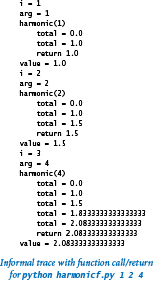### Informal function call/return trace.

One simple approach to following the control flow through function calls is to imagine that each function writes its name and argument(s) when it is called and its return value just before returning, with indentation added on calls and subtracted on returns. The result enhances the process of tracing a program by writing the values of its variables, which we have been using since Section 1.2. An informal trace for our example is shown at right.

### Basic terminology.

There are several concepts rolled up in the idea of a mathematical function and there are Python constructs corresponding to each, as summarized in this table: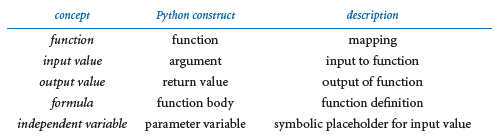When we use a symbolic name in a formula that defines a mathematical function (such as f(x) = 1 + x + x2), the symbol x is a placeholder for some input value that will be substituted into the formula to determine the output value. In Python, we use a parameter variable as a symbolic placeholder and we refer to a particular input value where the function is to be evaluated as an argument.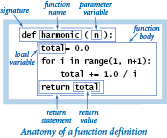### Function definition.

The first line of a function definition, known as its signature, gives a name to the function and to each parameter variable. The signature consists of the keyword `def`; the function name; a sequence of zero or more parameter variable names separated by commas and enclosed in parentheses; and a colon. The indented statements following the signature define the function body. The function body can consist of the kinds of statements that we discussed in Chapter 1. It also can contain a return statement, which transfers control back to the point where the function was called and returns the result of the computation or return value. The body may also define local variables, which are variables that are available only inside the function in which they are defined.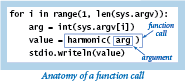### Function calls.

As we have seen throughout, a Python function call is nothing more than the function name followed by its arguments, separated by commas and enclosed in parentheses. Each argument can be an expression, which is evaluated and the resulting value passed as input to the function. When the function finishes, the return value takes the place of the function call as if it were the value of a variable (perhaps within an expression).

### Multiple arguments.

Like a mathematical function, a Python function can have more than one parameter variable, so it can be called with more than one argument.

### Multiple functions.

You can define as many functions as you want in a .py file. The functions are independent, except that they may refer to each other through calls. However, the definition of a function must appear before any global code that calls it. That is the reason that a typical Python program contains (1) `import` statements, (2) function definitions, and (3) arbitrary global code, in that order.

### Multiple return statements.

You can put `return` statements in a function wherever you need them: control goes back to the calling program as soon as the first `return` statement is reached.

### Single return value.

A Python function provides only one return value to the caller (or, more precisely, it returns a reference to one object). This policy is not as restrictive as it might seem, because Python data types can contain more information than a single number, boolean, or string. For example, you will see later in this section that you can use arrays as return values.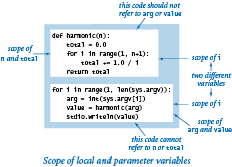### Scope.

The scope of a variable is the set of statements that can refer to that variable directly. The scope of a function's local and parameter variables is limited to that function; the scope of a variable defined in global code — known as a global variable — is limited to the `.py` file containing that variable. Therefore, global code cannot refer to either a function's local or parameter variables. Nor can one function refer to either the local or parameter variables that are defined in another function. When a function defines a local (or parameter) variable with the same name as a global variable (such as `i` in harmonicf.py), the variable name in the function refers to the local (or parameter) variable, not the global variable. While code in a function can refer to global variables, it should not do so: all communication from a caller to a function should take place via the function's parameter variables, and all communication from a function to its caller should take place via the function's return value.

### Default arguments.

A Python function may designate an argument to be optional by specifying a default value for that argument. If you omit an optional argument in a function call, then Python substitutes the default value for that argument. We have already encountered a few examples of this feature. You can specify an optional argument with a default value in a user-defined function by putting an equals sign followed by the default value after the parameter variable in the function signature. You can specify more than one optional argument in a function signature, but all of the optional arguments must follow all of the mandatory arguments. The `harmonic()` function in the table shown below uses a default argument.

### Side effects.

A pure function is a function that, given the same arguments, always returns the same value, without producing any observable side effects, such as consuming input, producing output, or otherwise changing the state of the system. In computer programming it is also useful to define functions that do produce side effects. In fact, we often define functions whose only purpose is to produce side effects. An explicit return statement is optional in such a function: control returns to the caller after Python executes the function's last statement. Functions with no specified return value actually return the special value `None`, which is usually ignored. The `drawTriangle()` function in the table shown below has side effects, and does not return a value.

### Type checking.

In mathematics, the definition of a function specifies both the domain and the range. For example, for the harmonic numbers, the domain is the positive integers and the range is the positive real numbers. In Python, we do not specify the types of the parameter variables or the type of the return value. As long as Python can apply all of the operations within a function, Python executes the function and returns a value. If Python cannot apply an operation to a given object because it is of the wrong type, it raises a run-time error to indicate the invalid type. This flexibility is a popular feature of Python (known as polymorphism) because it allows us to define a single function for use with objects of different types.

The table below summarizes our discussion by providing examples of function definitions.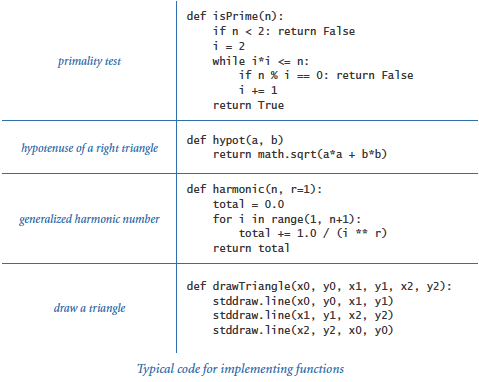## Implementing Mathematical Functions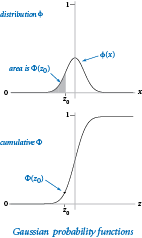We now consider two important functions that play an important role in science, engineering, and finance. The Gaussian (normal) distribution function is characterized by the familiar bell-shaped curve and defined by the formula: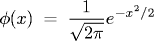and the cumulative Gaussian distribution function Φ(z) is defined to be the area under the curve defined by φ(x) above the x-axis and to the left of the vertical line x = z. Functions to calculate φ and Φ are not available in Python's `math` module, so we develop our own implementations.

### Closed form.

In the simplest situation, we have a closed-form mathematical formula defining our function in terms of functions that are implemented in Python's `math` module. This is the case for φ, so a function `pdf()` corresponding to the mathematical definition is easy to implement. For convenience, gauss.py uses the default arguments μ = 0 and σ = 1 and actually computes φ(x, μ, σ) = φ((x - μ) / σ) / σ

### No closed form.

If no formula is known, we may need a more complicated algorithm to compute function values. This situation is the case for Φ — no closed-form expression exists for this function. A Taylor series approximation to the ratio of Φ and φ turns out to be an effective basis for evaluating the function:
This formula readily translates to the Python code for the function `cdf()` in gauss.py. For small (respectively large) z, the value is extremely close to 0 (respectively 1), so the code directly returns 0 (respectively 1); otherwise, it uses the Taylor series to add terms until the sum converges. Again, for convenience, gauss.py actually computes Φ(z, μ, σ) = Φ((z - μ) / σ), using the defaults μ = 0 and σ = 1.

## Using Functions to Organize Code

With the ability to define functions, we can better organize our programs by defining functions within them when appropriate. For example, coupon.py is a version of couponcollector.py (from Section 1.4) that better separates the individual components of the computation:

• Given n, compute a random coupon value.
• Given n, do the coupon collection experiment.
• Get n from the command line, then compute and write the result.

Whenever you can clearly separate tasks within a computation, you should do so.

## Passing Arguments and Returning Values

Next, we examine the specifics of Python's mechanisms for passing arguments to and returning values from functions.

### Call by object reference.

You can use parameter variables anywhere in the body of the function in the same way as you use local variables. The only difference between a parameter variable and a local variable is that Python initializes the parameter variable with the corresponding argument provided by the calling code. We refer to this approach as call by object reference. One consequence of this approach is that if a parameter variable refers to a mutable object and you change that object's value within a function, then this also changes the object's value in the calling code (because it is the same object). Next, we explore the ramifications of this approach.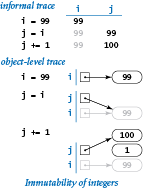### Immutability and aliasing.

As discussed in Section 1.4, arrays are mutable data types, because we can change array elements. By contrast, a data type is immutable if it is not possible to change the value of an object of that type. The other data types that we have been using (`int`, `float`, `str`, and `bool`) are all immutable. In an immutable data type, operations that might seem to change a value actually result in the creation of a new object, as illustrated in the simple example at right. First, the statement `i = 99` creates an integer 99, and assigns to `i` a reference to that integer. Then `j = i` assigns `i` (an object reference) to `j`, so both `i` and `j` reference the same object — the integer 99. Two variables that reference the same object are said to be aliases. Next, `j += 1` results in `j` referencing an object with value 100, but it does not do so by changing the value of the existing integer from 99 to 100! Indeed, since `int` objects are immutable, no statement can change the value of that existing integer. Instead, that statement creates a new integer 1, adds it to the integer 99 to create another new integer 100, and assigns to `j` a reference to that integer. But `i` still references the original 99. Note that the new integer 1 has no reference to it in the end — that is the system's concern, not ours.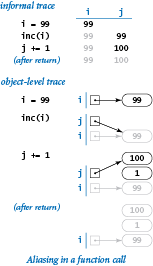### Integers, floats, booleans, and strings as arguments.

Whenever you pass arguments to a function, the arguments and the function's parameter variables become aliases. In practice, this is the predominant use of aliasing in Python. For purposes of illustration, suppose that we need a function that increments an integer (our discussion applies to any more complicated function as well). A programmer new to Python might try this definition:

 ```def inc(j): j += 1 ```

and then expect to increment an integer `i` with the call `inc(i)`. Code like this would work in some programming languages, but it has no effect in Python, as shown in the figure at right.

To increment variable `i`, we could use the definition

 ```def inc(j): j += 1 return j ```

and call the function with the assignment statement `i = inc(i)`.

The same holds true for any immutable type. A function cannot change the value of an integer, a float, a boolean, or a string.

### Arrays as arguments.

When a function takes an array as an argument, it implements a function that operates on an arbitrary number of objects. For example, the following function computes the mean (average) of an array of floats or integers:

 ```def mean(a): total = 0.0 for v in a: total += v return total / len(a) ```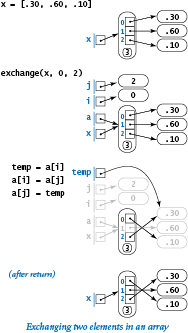### Side effects with arrays.

Since arrays are mutable, it is often the case that the purpose of a function that takes an array as argument is to produce a side effect (such as changing the order of array elements). A prototypical example of such a function is one that exchanges the elements at two given indices in a given array. We can adapt the code that we examined at the beginning of Section 1.4:

 ```def exchange(a, i, j): temp = a[i] a[i] = a[j] a[j] = temp ```

A formal trace of a call on this function is shown on the right.

A second prototypical example of a function that takes an array argument and produces side effects is one that randomly shuffles the elements in the array, using the version of the algorithm that we examined in Section 1.4 (and the `exchange()` function just defined):

 ```def shuffle(a): n = len(a) for i in range(n): r = random.randrange(i, n) exchange(a, i, r) ```

### Arrays as return values.

A function can return an array. For example, consider the following function, which returns an array of random floats:

 ```def randomarray(n): a = stdarray.create1D(n, 0.0) for i in range(n): a[i] = random.random() return a ```

The table below concludes our discussion of arrays as function arguments by highlighting some typical array-processing functions.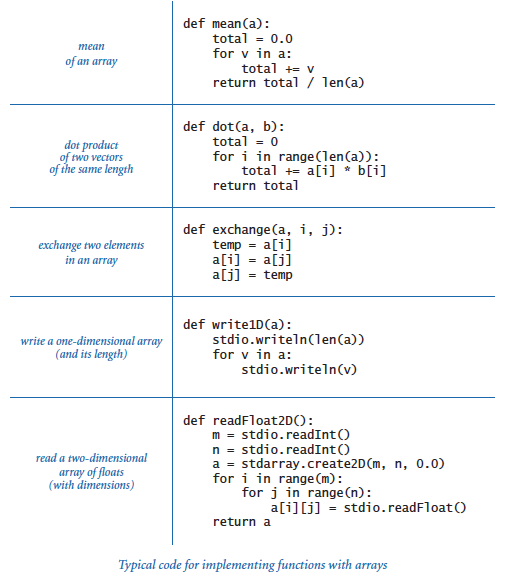## Superposition of Sound WavesNotes like concert A have a pure sound that is not very musical, because the sounds that you are accustomed to hearing have many other components. Most musical instruments produce harmonics (the same note in different octaves and not as loud), or you might play chords (multiple notes at the same time). To combine multiple sounds, we use superposition: simply add their waves together and rescale to make sure that all values stay between -1 and +1.

The program playthattunedeluxe.py is a version of playthattune.py (from Section 1.5) that encapsulates the sound wave calculation and adds harmonics. Try running playthattunedeluxe.py repeatedly with its standard input redirected to each of these data files (created by various students): elise.txt, ascale.txt, stairwaytoheaven.txt, entertainer.txt, firstcut.txt, freebird.txt, and looney.txt.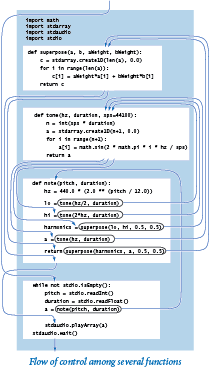#### Q & A

Q. Can I use the statement return in a function without specifying a value?

A. Yes. Technically, it returns the `None` object, which is the sole value of the type `NoneType`.

Q. What happens if a function has one control flow that leads to a `return` statement that returns a value but another control flow that reaches the end of the function body?

A. It would be poor style to define such a function, because doing so would place a severe burden on the function's callers: the callers would need to know under which circumstances the function returns a value, and under which circumstances it returns `None`.

Q. What happens if I compose code in the body of a function that appears after the `return` statement?

A. Once a `return` statement is reached, control returns to the caller. So any code in the body of a function that appears after a return statement is useless; it is never executed. In Python, it is poor style, but not illegal to define such a function.

Q. What happens if I define two functions with the same name (but possibly a different number of arguments) in the same `.py` file?

A. This is known as function overloading, which is embraced by many programming languages. Python, however, is not one of those languages: the second function definition will overwrite the first one. You can often achieve the same effect by using default arguments.

Q. What happens if I define two functions with the same name in different files?

A. That is fine. For example, it would be good design to have a function named `pdf()` in gauss.py that computes the Gaussian probability density function and another function named `pdf()` in `cauchy.py` that computes the Cauchy probability density function. In Section 2.2 you will learn how to call functions defined in different `.py` files.

Q. Can a function change the object to which a parameter variable is bound?

A. Yes, you can use a parameter variable on the left side of an assignment statement. However, many Python programmers consider it poor style to do so. Note that such an assignment statement has no effect in the client.

Q. The issue with side effects and mutable objects is complicated. Is it really all that important?

A. Yes. Properly controlling side effects is one of a programmer's most important tasks in large systems. Taking the time to be sure that you understand the difference between passing arrays (which are mutable) and passing integers, floats, booleans, and strings (which are immutable) will certainly be worthwhile. The very same mechanisms are used for all other types of data, as you will learn in Chapter 3.

Q. How can I arrange to pass an array to a function in such a way that the function cannot change the elements in the array?

A. There is no direct way to do so. In Section 3.3 you will see how to achieve the same effect by building a wrapper data type and passing an object of that type instead. You will also see how to use Python's built-in `tuple` data type, which represents an immutable sequence of objects.

Q. Can I use a mutable object as a default value for an optional argument?

A. Yes, but it may lead to unexpected behavior. Python evaluates a default value only once, when the function is defined (not each time the function is called). So, if the body of a function modifies a default value, subsequent function calls will use the modified value. Similar difficulties arise if you initialize the default value by calling an impure function. For example, after Python executes the code fragment

 ```def append(a=[], x=random.random()): a += [x] return a b = append() c = append() ```

`b[]` and `c[]` are aliases for the same array of length 2 (not 1), which contains one float repeated twice (instead of two different floats).

#### Exercises

1. Compose a function `max3()` that takes three `int` or `float` arguments and returns the largest one.

Solution:

```def max3(a, b, c)
max = a
if b > max:
max = b
if c > max:
max = c
return max
```
2. Compose a function `odd()` that takes three `bool` arguments and returns `True` if an odd number of arguments are `True`, and `False` otherwise.

3. Compose a function `majority()` that takes three `bool` arguments and returns `True` if at least two of the arguments are `True`, and `False` otherwise. Do not use an `if` statement.

Solution: Here are two solutions. The first is concise. The second is silly, but does adhere to the rules.

 ```def majority(a, b, c): return (a and b) or (a and c) or (b and c) def majority(a, b, c): while a and b: return True while a and c: return True while b and c: return True return False ```
4. Compose a function `areTriangular()` that takes three numbers as arguments and returns `True` if they could be the sides of a triangle (none of them is greater than or equal to the sum of the other two), and False otherwise.

5. Compose a function `sigmoid()` that takes a `float` argument `x` and returns the `float` obtained from the formula: 1 / (1 - e-x).

6. Compose function `lg()` that takes an integer `n` as an argument and returns the base-2 logarithm of `n`. You may use Python's `math` module.

7. Compose a function `lg()` that takes an integer `n` as an argument and returns the largest integer not larger than the base-2 logarithm of `n`. Do not use the standard Python `math` module.

8. Compose a function `signum()` that takes a `float` argument `n` and returns -1 if `n` is less than 0, 0 if `n` is equal to 0, and +1 if `n` is greater than 0.

9. Consider this function `duplicate()`:

 ```def duplicate(s): t = s + s ```

What does the following code fragment write?

 ```s = 'Hello' s = duplicate(s) t = 'Bye' t = duplicate(duplicate(duplicate(t))) stdio.writeln(s + t) ```
10. Consider this function `cube()`:

```def cube(i):
i = i * i * i
```

How many times is the following `while` loop iterated?

```i = 0
while i < 1000:
cube(i)
i += 1
```

Solution: Just 1000 times. A call to `cube()` has no effect on client code. It changes the value of its local parameter variable `i`, but that change has no effect on the `i` in the `while` loop, which is a different variable. If you replace the call to `cube(i)` with the statement `i = i * i * i` (maybe that was what you were thinking), then the loop is iterated five times, with `i` taking on the values 0, 1, 2, 9, and 730 at the beginning of the five iterations.

11. What does the following code fragment write?

 ```for i in range(5): stdio.write(i) for j in range(5): stdio.write(i) ```

Solution: 0123444444. Note that the second call to `stdio.write()` uses `i`, not `j`. Unlike analogous loops in many other programming languages, when the first for loop terminates, the variable `i` is 4 and it remains in scope.

12. The following checksum formula is widely used by banks and credit card companies to validate legal account numbers:

 d0 + f(d1) + d2 + f(d3) + d4 + f(d5) + d6 + ... = 0 (mod 10)

The di are the decimal digits of the account number and f(d) is the sum of the decimal digits of 2d (for example, f(7) = 5 because 2 × 7 = 14 and 1 + 4 = 5). For example, 17327 is valid because 1 + 5 + 3 + 4 + 7 = 20, which is a multiple of 10. Implement the function f and compose a program to take a 10-digit integer as a command-line argument and print a valid 11-digit number with the given integer as its first 10 digits and the checksum as the last digit.

13. Given two stars with angles of declination and right ascension (d1, a1) and (d2, a2), the angle they subtend is given by the Haversine formula:

 2 arcsin((sin2(d/2) + cos(d1) cos(d2) sin2(a/2))1/2)

where a1 and a2 are angles between -180 and 180 degrees, d1 and d2 are angles between -90 and 90 degrees, a = a2 - a1, d = d2 - d1. Compose a program to take declination and right ascension of two stars as command-line arguments and write the angle they subtend. Hint: Be careful about converting from degrees to radians.

See the similar exercise from Section 1.2. Latitude corresponds to declination and longitude to ascension.

14. Compose a `readBoolean2D()` function that reads a two-dimensional matrix of 0 and 1 values (with dimensions) into an array of booleans.

Solution: The body of the function is virtually the same as for the corresponding function given in the table earlier in this page for two-dimensional arrays of floats:
 ```def readBool2D(): m = stdio.readInt() n = stdio.readInt() a = stdarray.create2D(m, n, False) for i in range(m): for j in range(n): a[i][j] = stdio.readBool() return a ```
15. Compose a function that takes an array `a[]` of strictly positive floats as its argument and rescales the array so that each element is between 0 and 1 (by subtracting the minimum value from each element and then dividing each element by the difference between the minimum and maximum values). Use the built-in `max()` and `min()` functions.

16. Compose a function `histogram()` that takes an array `a[]` of integers and an integer `m` as arguments and returns an array of length `m` whose `i`th entry is the number of times the integer `i` appears in the argument array. Assume that the values in `a[]` are all between 0 and `m`-1, so that the sum of the values in the returned array should be equal to `len(a)`.

17. Assemble code fragments in this section and in Section 1.4 to develop a program that takes an integer `n` from the command line and writes `n` five-card hands, separated by blank lines, drawn from a randomly shuffled card deck, one card per line using card names like `Ace of Clubs`.

18. Compose a function `multiply()` that takes two square matrices of the same dimension as arguments and returns their product (another square matrix of that same dimension). Extra credit: Make your program work whenever the number of columns in the first matrix is equal to the number of rows in the second matrix.

19. Compose a function `any()` that takes an array of booleans as its argument and returns `True` if any of the entries in the array is `True`, and `False` otherwise. Compose a function `all()` that takes an array of booleans as its argument and returns `True` if all of the entries in the array are `True`, and `False` otherwise. Note that `all()` and `any()` are Python built-in functions; the goal of this exercise is to understand them better by creating your own versions.

20. Develop a version of `getCoupon()` that better models the situation when one of the coupons is rare: choose one value at random, return that value with probability 1/(1000n), and return all other values with equal probability. Extra credit: How does this change affect the average value of the coupon collector function?

21. Modify playthattune.py (from Section 1.5) to add harmonics two octaves away from each note, with half the weight of the one-octave harmonics.

#### Creative Exercises

1. Birthday problem. Compose a program with appropriate functions for studying the birthday problem (see the related exercise in Section 1.4).

2. Euler's totient function. Euler's totient function is an important function in number theory: φ(n) is defined as the number of positive integers less than or equal to n that are relatively prime with n (no factors in common with n other than 1). Compose a function that takes an integer argument n and returns φ(n). Include global code that takes an integer from the command line, calls the function, and writes the result.

3. Harmonic numbers. Compose a program `harmonic.py` that defines three functions `harmonic()`, `harmonicSmall()`, and `harmonicLarge()` for computing the harmonic numbers. The `harmonicSmall()` function should just compute the sum (as in harmonic.py), the `harmonicLarge()` function should use the approximation Hn = loge(n) + γ + 1/(2n) - 1/(12n2) + 1/(120n4) (the number γ = .577215664901532... is known as Euler's constant), and the `harmonic()` function should call `harmonicSmall()` for n < 100 and `harmonicLarge()` otherwise.

4. Gaussian random values. Experiment with the following functon for generating random variables from the Gaussian distribution, which is based on generating a random point in the unit circle and using a form of the Box-Muller transform. (See the "Gaussian random numbers" exercise at the end of Section 1.2.)

 ```def gaussian(): r = 0.0 while (r >= 1.0) or (r == 0.0): x = random.uniform(-1.0, 1.0) y = random.uniform(-1.0, 1.0) r = x*x + y*y return x * math.sqrt(-2.0 * math.log(r) / r) ```

Take a command-line argument `n` and generate `n` random numbers, using an array `a[]` of 20 integers to count the numbers generated that fall between `i*.05` and `(i+1)*.05` for `i` from 0 to 19. Then use `stddraw` to plot the values and to compare your result with the normal bell curve. Remark: This approach is faster and more accurate than the one described in the "Gaussian random numbers" exercise in Section 1.2. Although it involves a loop, the loop is executed only 4/π (about 1.273) times on average. This reduces the overall expected number of calls to transcendental functions.

5. Binary search. A general method that we study in detail in Section 4.2 is effective for computing the inverse of a cumulative probability density function like `cdf()`. Such functions are continuous and nondecreasing from (0, 0) to (1, 1). To find the value x0 for which f(x0) = y0, check the value of f(.5). If it is greater than y0, then x0 must be between 0 and .5; otherwise, it must be between .5 and 1. Either way, we halve the length of the interval known to contain x0. Iterating, we can compute x0 to within a given tolerance. Add a function `cfdInverse()` to gauss.py that uses binary search to compute the inverse.

Solution: See gaussinv.py.

6. Black-Scholes option valuation. The Black Scholes formula supplies the theoretical value of a European call option on a stock that pays no dividends, given the current stock price s, the exercise price x, the continuously compounded risk-free interest rate r, the standard deviation σ of the stock's return (volatility) and the time (in years) to maturity t. The value is given by the formula sΦ(a) - xe-rtφ(b), where Φ(z) is the Gaussian cumulative distribution function, a = (ln(s/x) + (r + σ2/2)t)/(σt1/2), and b = a - σt1/2. Compose a program that takes `s`, `x`, `r`, `sigma`, and `t` from the command line and writes the Black-Scholes value. For now, copy the definitions of the `phi()` and `Phi()` functions from gauss.py into your program. In the next section of the website, you'll learn how to define a function in one `.py` file such that it can be called by the code in another `.py` file.

Myron Scholes won the 1997 Nobel Prize in Economics for the Black-Scholes paper.

Solution: See blackscholes.py.

7. Implied volatility. Typically the volatility is the unknown value in the Black-Scholes formula. Write a program that reads `s`, `x`, `r`, `t`, and the current price of the option from the command line and uses binary search (see a previous exercise in this section) to compute σ.

8. Horner's method. Compose a program with a function `evaluate(x, a)` that evaluates the polynomial a(x) whose coefficients are the elements in the array `a[]`:

 a0 + a1x1 + a2x2 + ... + an-2xn-2 + an-1xn-1

Use Horner's method, an efficient way to perform the computations that is suggested by the following parenthesization:

 a0 + x (a1 + x( a2 + ... + x(an-2 + xan-1))...)

Then compose a function `exp()` that calls `evaluate()` to compute an approximation to ex, using the first n terms of the Taylor series expansion ex = 1 + x + x2/2! + x3/3! + ... Take an argument `x` from the command line, and compare your result against that computed by `math.exp(x)`.

Include code to check your answer against that computed by `math.exp()`.

Solution: See horner.py.

9. Benford's law. The American astronomer Simon Newcomb observed a quirk in a book that compiled logarithm tables: the beginning pages were much grubbier than the ending pages. He suspected that scientists performed more computations with numbers starting with 1 than with 8 or 9, and postulated the first digit law, which says that under general circumstances, the leading digit is much more likely to be 1 (roughly 30%) than the digit 9 (less than 4%). This phenomenon is known as Benford's law and is now often used as a statistical test. For example, IRS forensic accountants rely on it to discover tax fraud. Compose a program that reads in a sequence of integers from standard input and tabulates the number of times each of the digits 1-9 is the leading digit, breaking the computation into a set of appropriate functions. Use your program to test the law on some tables of information from your computer or from the web. Then, compose a program to foil the IRS by generating random amounts from \$1.00 to \$1,000.00 with the same distribution that you observed.

The file princeton-files.txt is a list of file sizes on a public Unix machine at Princeton. Try running your program on that file.

Solution: See benford.py.

10. Binomial distribution. Compose a function `binomial()` that accepts an integer `n`, an integer `k`, and a float `p`, and computes the probability of obtaining exactly `k` heads in `n` biased coin flips (heads with probability `p`) using the formula

 f(k, n, p) = pk(1 - p)n-kn! / (k!(n-k)!)

Hint: To avoid computing with huge integers, compute x = ln f(k, n, p) and then return ex. In the global code, take `n` and `p` from the command line and check that the sum over all values of `k` between 0 and `n` is (approximately) 1. Also, compare every value computed with the normal approximation

 f(k, n, p) ≈ Φ(k + 1/2, np, (np(1-p)1/2) - Φ(k - 1/2, np, (np(1-p)1/2)
11. Coupon collecting from a binomial distribution. Compose a version of `getCoupon()` that uses `binomial()` from the previous exercise to return coupon values according to the binomial distribution with p = 1/2. Hint: Generate a uniformly distributed random number x between 0 and 1, then return the smallest value of k for which the sum of f(n, j, p) for all j < k exceeds x. Extra credit: Develop a hypothesis for describing the behavior of the coupon collector function under this assumption.

12. Chords. Compose a version of playthattunedeluxe.py that can handle songs with chords (including harmonics). Develop an input format that allows you to specify different durations for each chord and different amplitude weights for each note within a chord. Create test files that exercise your program with various chords and harmonics, and create a version of Fur Elise that uses them.

13.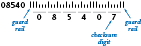Postal bar codes. The POSTNET barcode used by the U.S. Postal System to route mail is defined as follows: Each decimal digit in the zip code is encoded using a sequence of three half-height and two full-height bars. The barcode starts and ends with a full-height bar (the guard rail) and includes a checksum digit (after the five-digit zip code or ZIP+4), computed by summing up the original digits modulo 10. Implement the following functions:

• Draw a half-height or full-height bar on `stddraw`.
• Given a digit, draw its sequence of bars.
• Compute the checksum digit.

and a test client that reads in a five- (or nine-) digit ZIP code as the command-line argument and draws the corresponding postal bar code.

 Value Encoding 0 1 2 3 4 5 6 7 8 9 `||╷╷╷` `╷╷╷||` `╷╷|╷|` `╷╷||╷` `╷|╷╷|` `╷|╷|╷` `╷||╷╷` `|╷╷╷|` `|╷╷|╷` `|╷|╷╷`
14. Calendar. Compose a program that takes two command-line arguments `m` and `y` and writes the monthly calendar for the `m`th month of year `y`, as in this example:

 ``` February 2009 S M Tu W Th F S 1 2 3 4 5 6 7 8 9 10 11 12 13 14 15 16 17 18 19 20 21 22 23 24 25 26 27 28 ```

Hint: See leapyear.py and the "Day of the week" exercise in Section 1.2.

Solution: See calendar.py.

15. Fourier spikes. Compose a program that takes a command-line argument n and plots the function:

 (cos(t) + cos(2t) + cos(3t) + cos(4t) + ... + cos(nt)) / n
for 500 equally spaced samples of t from -10 to 10 (in radians). Run your program for n = 5 and n = 500. Note: You will observe that the sum converges to a spike (0 everywhere except a single value). This property is the basis for a proof that any smooth function can be expressed as a sum of sinusoids.